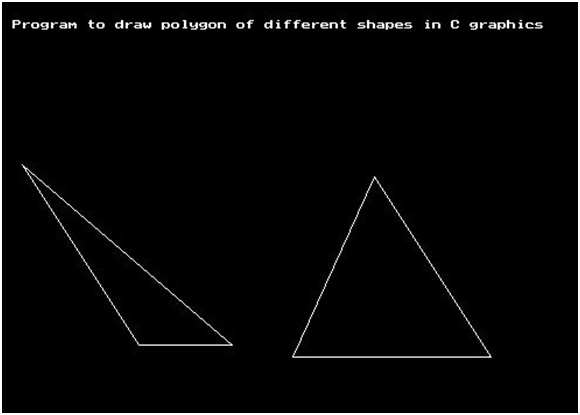# Design polygons of various shapes using graphics.h in C

In this article, we are going to learn about the drawpoly() function of graphics.h header file and use them to create polygon design of different shapes.
Submitted by Manu Jemini, on March 21, 2018

Polygon function accepts an array of numbers, which should be even as these numbers are the co-ordinates of different vertices of the shape.

The First and the last co-ordinate means the first two and the last two numbers should be repeated to complete the shape, as every polygon is close.

This function is can be used to create any polygon as it asks for two parameters to be passed.

First is the number of co-ordinates, which as we have already talked about defines the location of the vertices.

Seconds is the array which contains all these numbers, defines the pairs of points on x and y-axis.

These functions are from the header file graphics.h and should be included.

## graphics.h - drawpoly() function example in C

﻿

```#include <graphics.h>
#include <conio.h>

int main()
{
//initilizing graphic driver and
//graphic mode variable
int graphicdriver=DETECT,graphicmode;

//calling initgraph with parameters
initgraph(&graphicdriver,&graphicmode,"c:\\turboc3\\bgi");

//Printing message for user
outtextxy(10, 10 + 10, "Program to draw polygon of different shapes in C graphics");

//points of ploygon 1
int p1[]={320,150,420,300,500,300,320,150};

//points of ploygon
int p2[]={320,150,420,300,250,300,320,150};

//drawing polygon 1
drawpoly(4, p1);

//drawing polygon 2
drawpoly(4, p2);

getch();

return 0;
}
```

Output